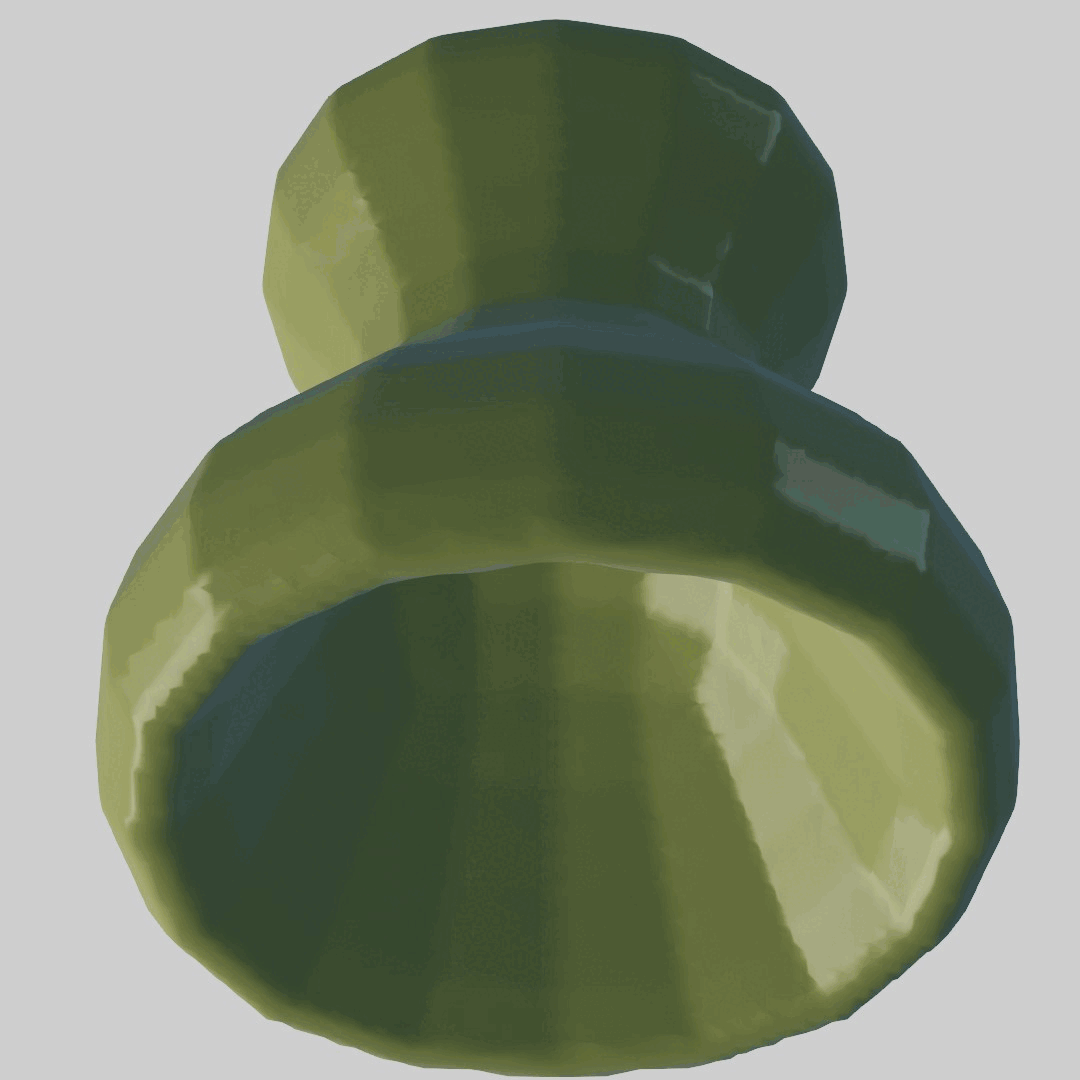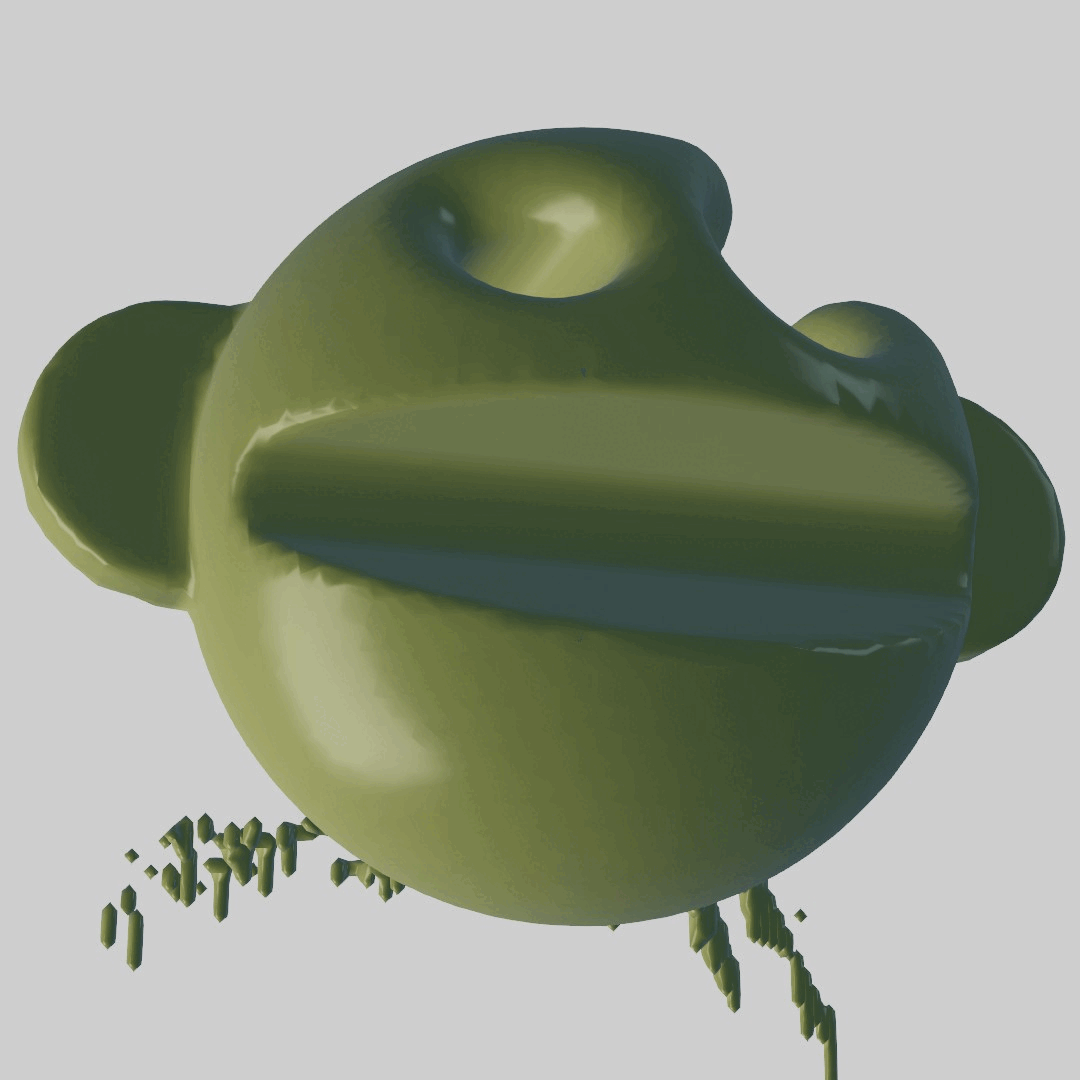# 3D Mesh Interpolation

Shiva Peri, Nick Diamant, Himalini Gururaj - Final Project

## Objective

The motivation for this projects follows from these papers: Learning Smooth Neural Functions via Lipschitz Regularization, and DeepSDF: Learning Continuous Signed Distance Functions for Shape Representation. We wanted to apply the interpolation techniques described to arbitary input meshes. As artists, we were curious what meshes would potentially 'break' these interpolation methods.

## Method 1: Learning Approach

#### Architecture

The learning based approach involves using the DeepSDF architecture with Lipschitz regularization. The key insight into this approach is understanding how neural fields are encoded as signed distance functions (SDFs). In the left image below, we can see that an input latent vector (blue) concatenated with an input query point (gray) is fed into the DeepSDF network. The output SDF (yellow) returns a float value representing how close or far from the surface the query point is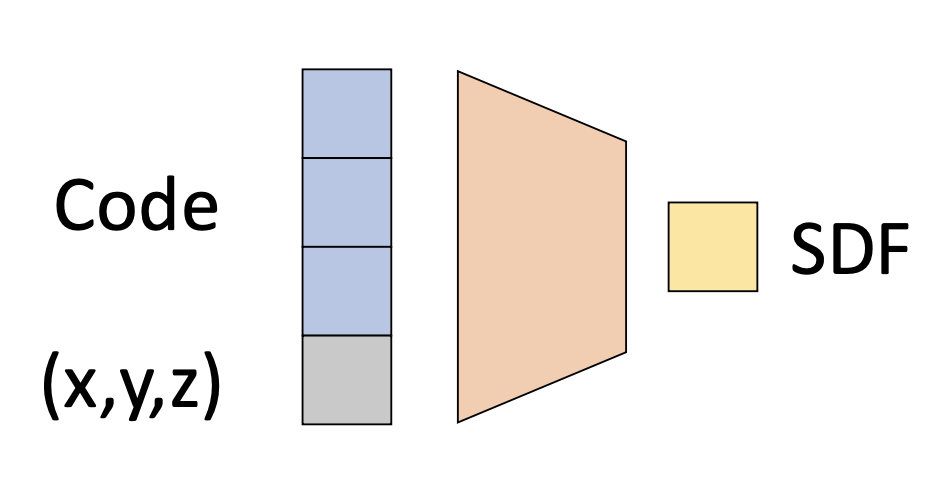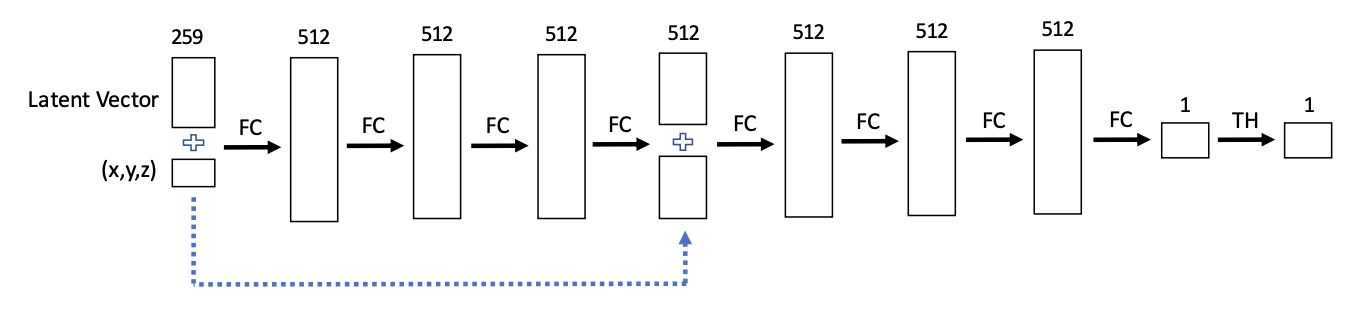The right image above shows the DeepSDF architecture. In our implementation, we used five fully connected layers with TanH activations, LayerNorm, and Lipschitz regularization. While the DeepSDF paper notes that using a random latent vector which is optimized during training provides better results, we used the one-hot encoding. That is, given let's say three input meshes (A, B, C), the corresponding latent vectors would be [1, 0, 0], [0, 1, 0], [0, 0, 1]
We used MSELoss between the ground truth SDF and our network's predicted SDF. Additionally, we added in a Lipschitz regularization term for interpolation stability.

#### Reconstruction Results

The first task we attempted was a simple reconstruction of two input meshes. As a result, out latent vector dimension is 2, with the input vector having dimension 2 + 3 = 5, including the query point. Below, we display the input mesh and the ground truth point cloud representation of the input mesh. Note in the point cloud visualizations, red points denote positive signed distances from the surface and blue points denote negative signed distances.

The right two columns are our reconstruction results. The point cloud (PC) reconstruction shows a grainier version of the ground truth. From the latent SDF, we used marching cubes (MC) to reconstruct a mesh, which we rendered in Blender.

Input Mesh
Ground Truth PC
PC Reconstruction
MC Reconstruction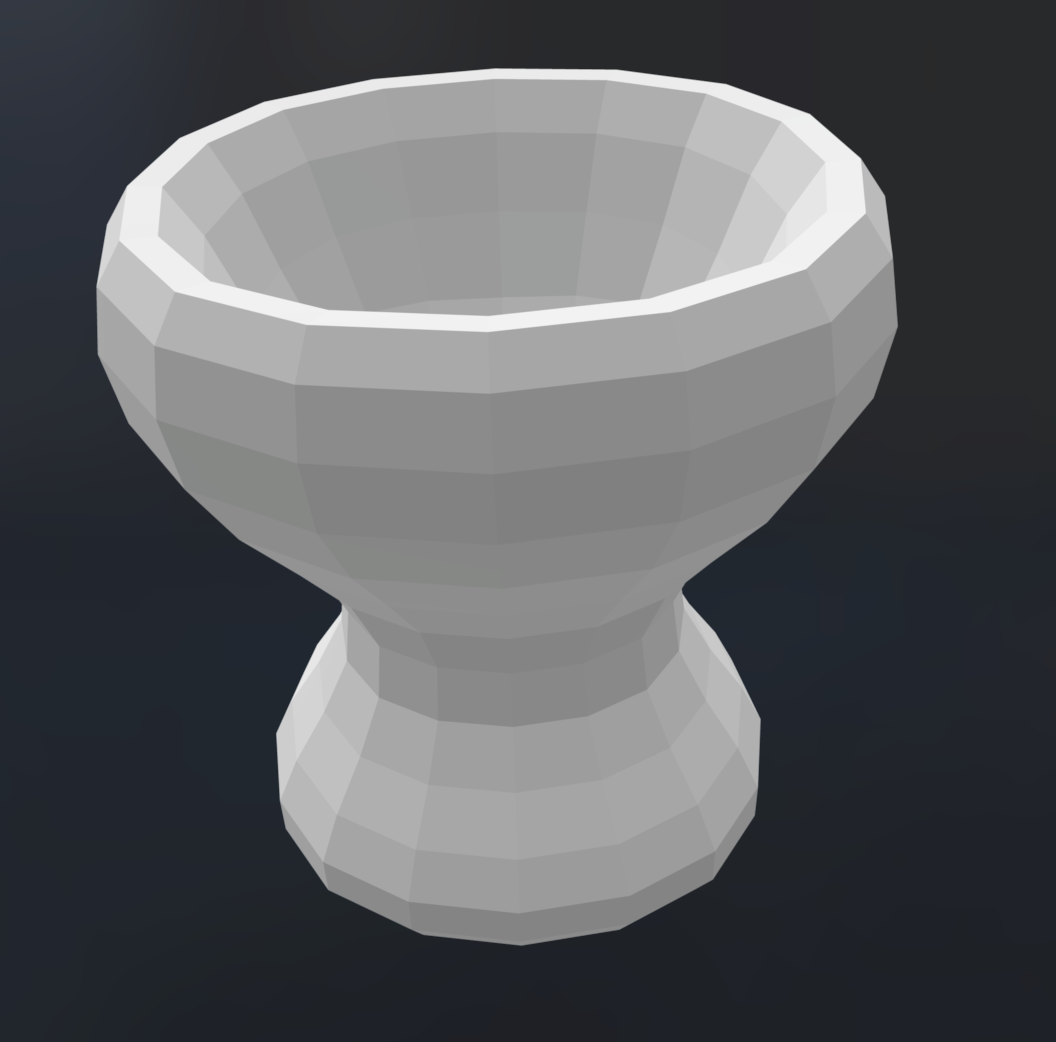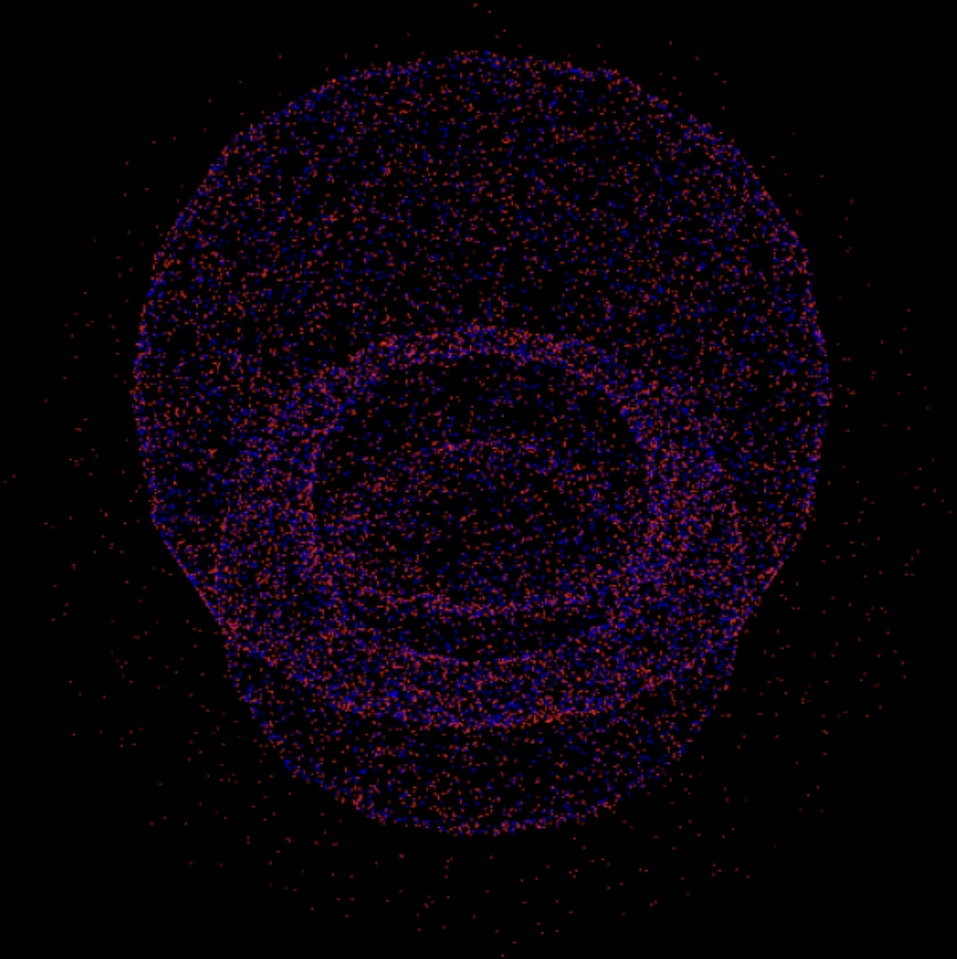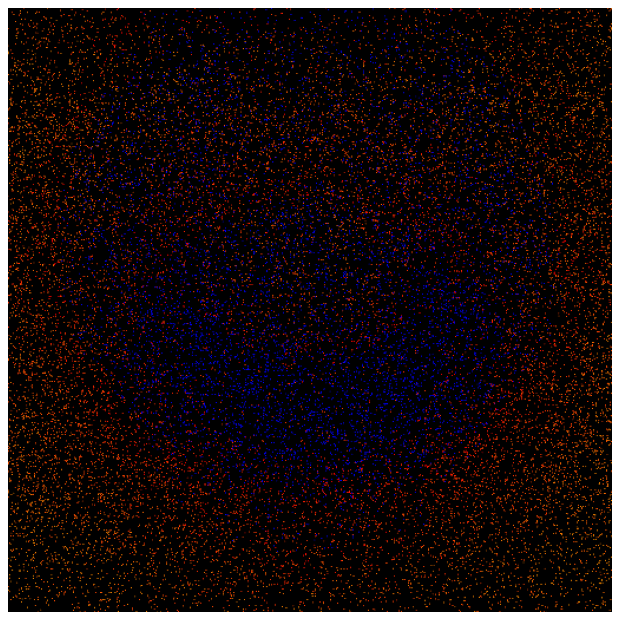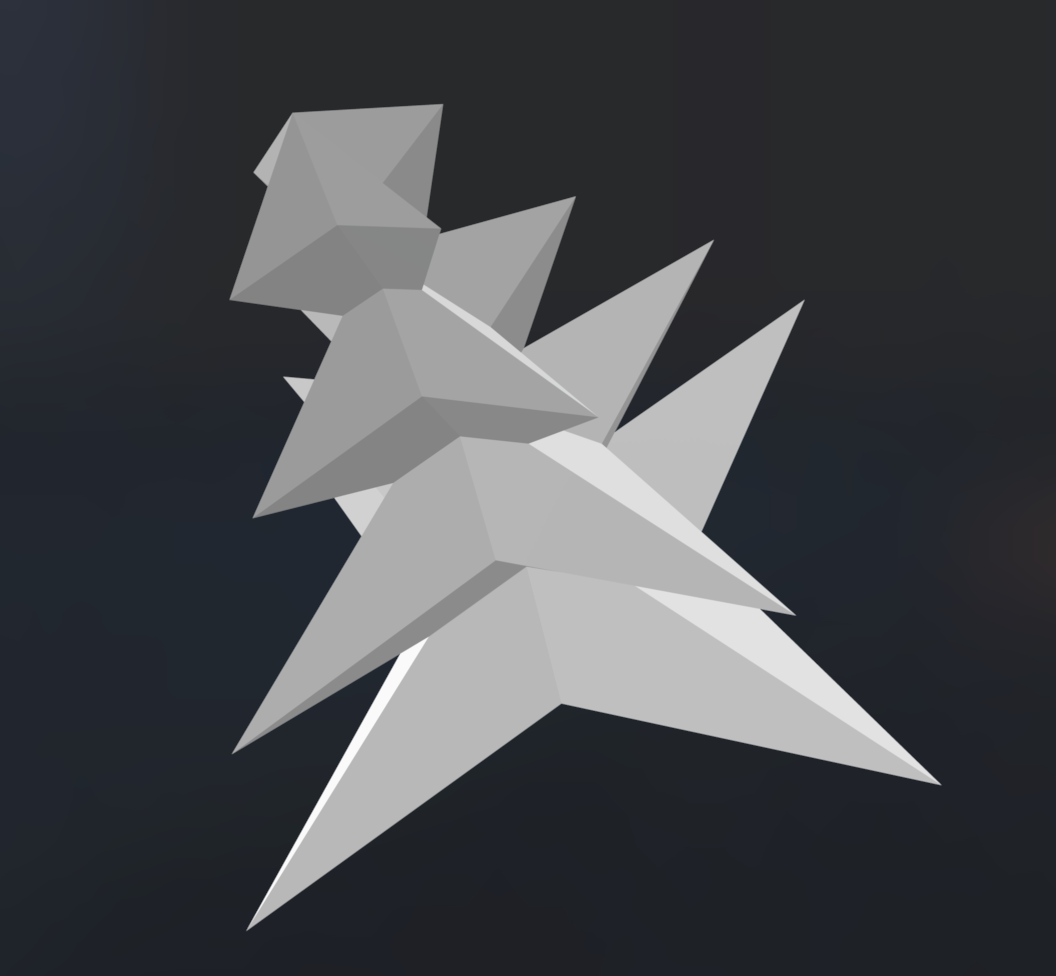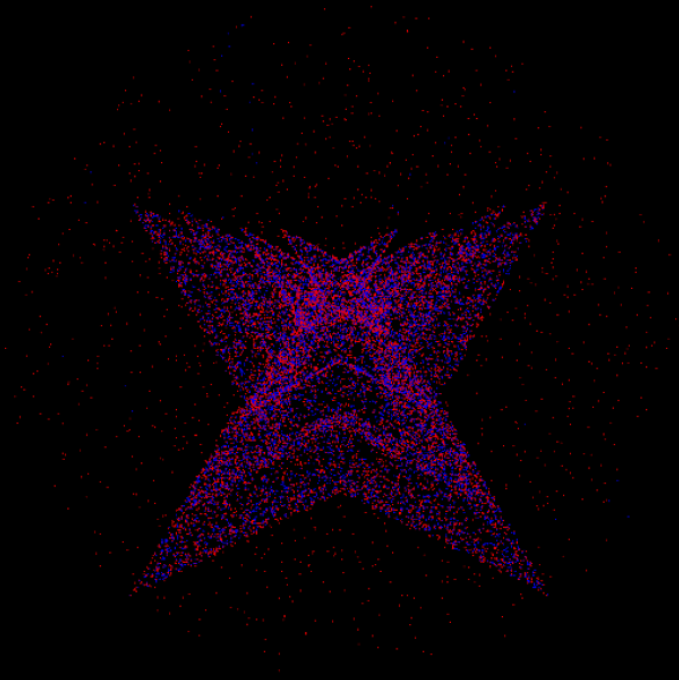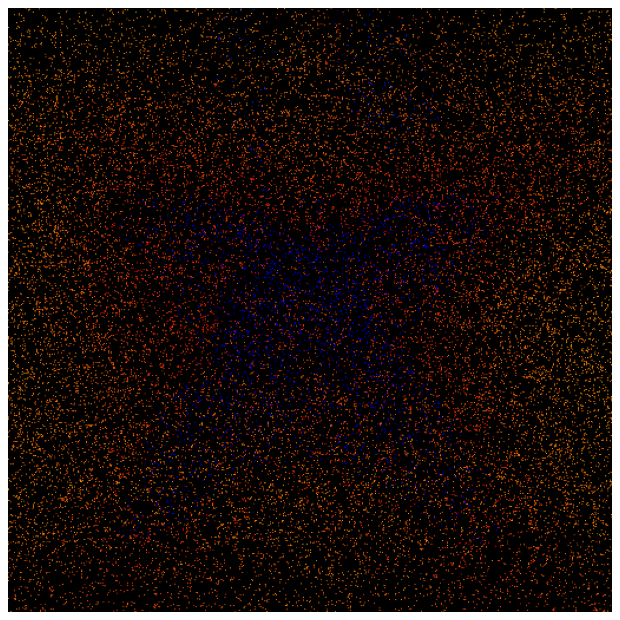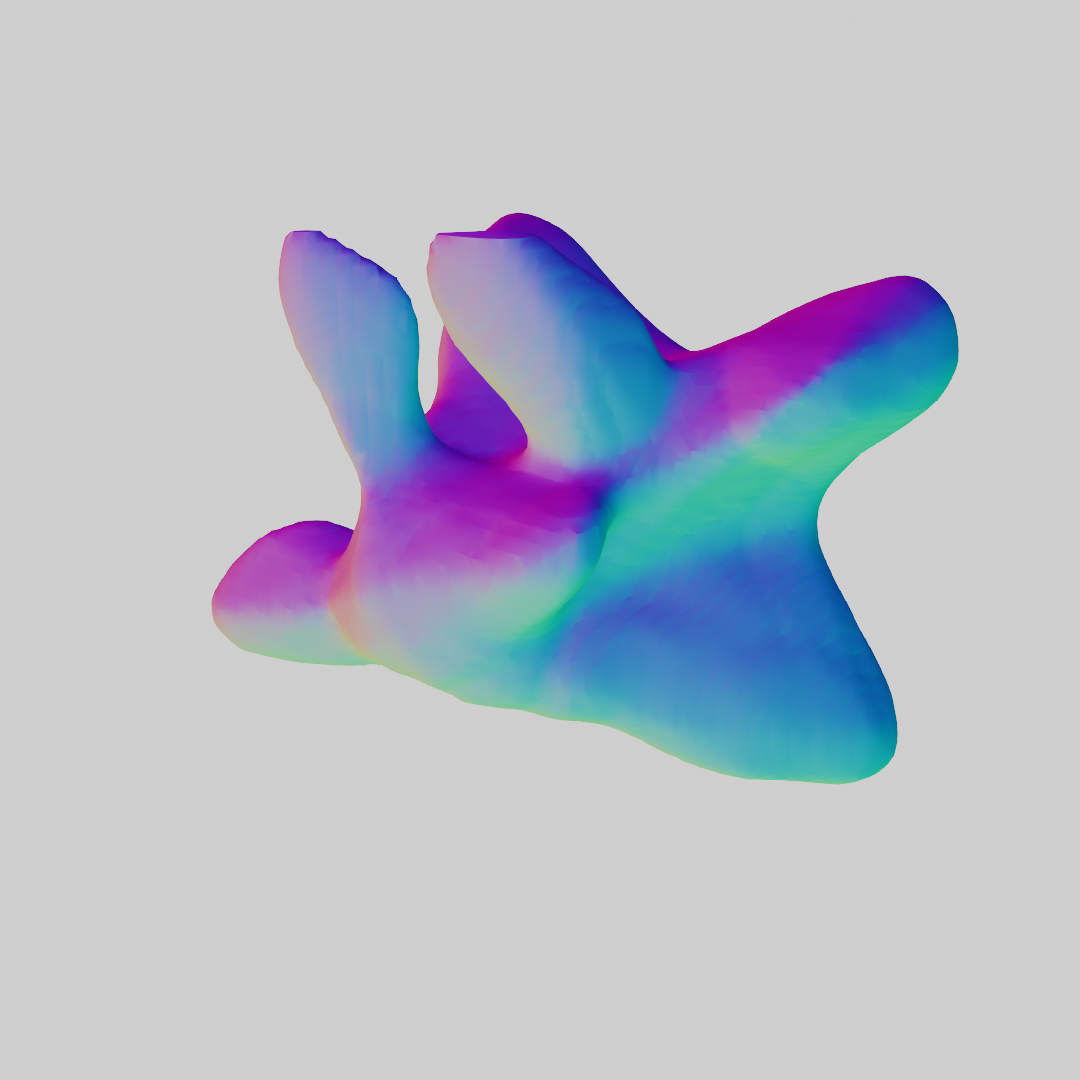#### Interpolation Results

Following from the above reconstructions, we used the same input meshes to describe interpolations. We denoted the bowl mesh as [1, 0] and the spiky mesh as [0, 1]. Below we visualize various interpolations in the latent space. The left depicts a linear interpolation between the direct encodings of the two meshes. The middle image depicts an interpolation from the opposite corners of the latent space. The right image depicts a circular path centered at [.5, .5] with radius .5. Both the right two images demonstrate the smoothness of the latent space, even with extrapolated latent values.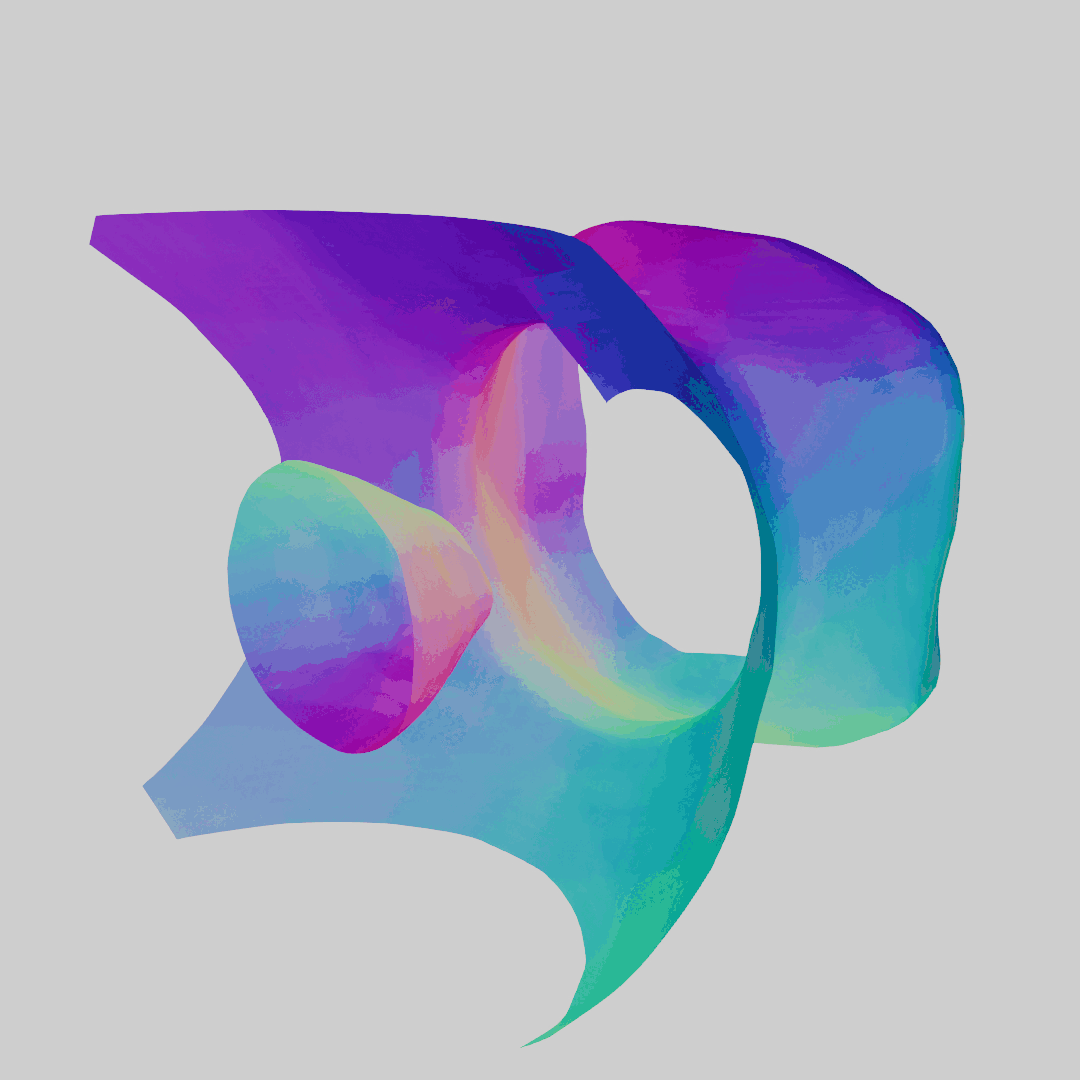Lerp from [1, 0] to [0, 1]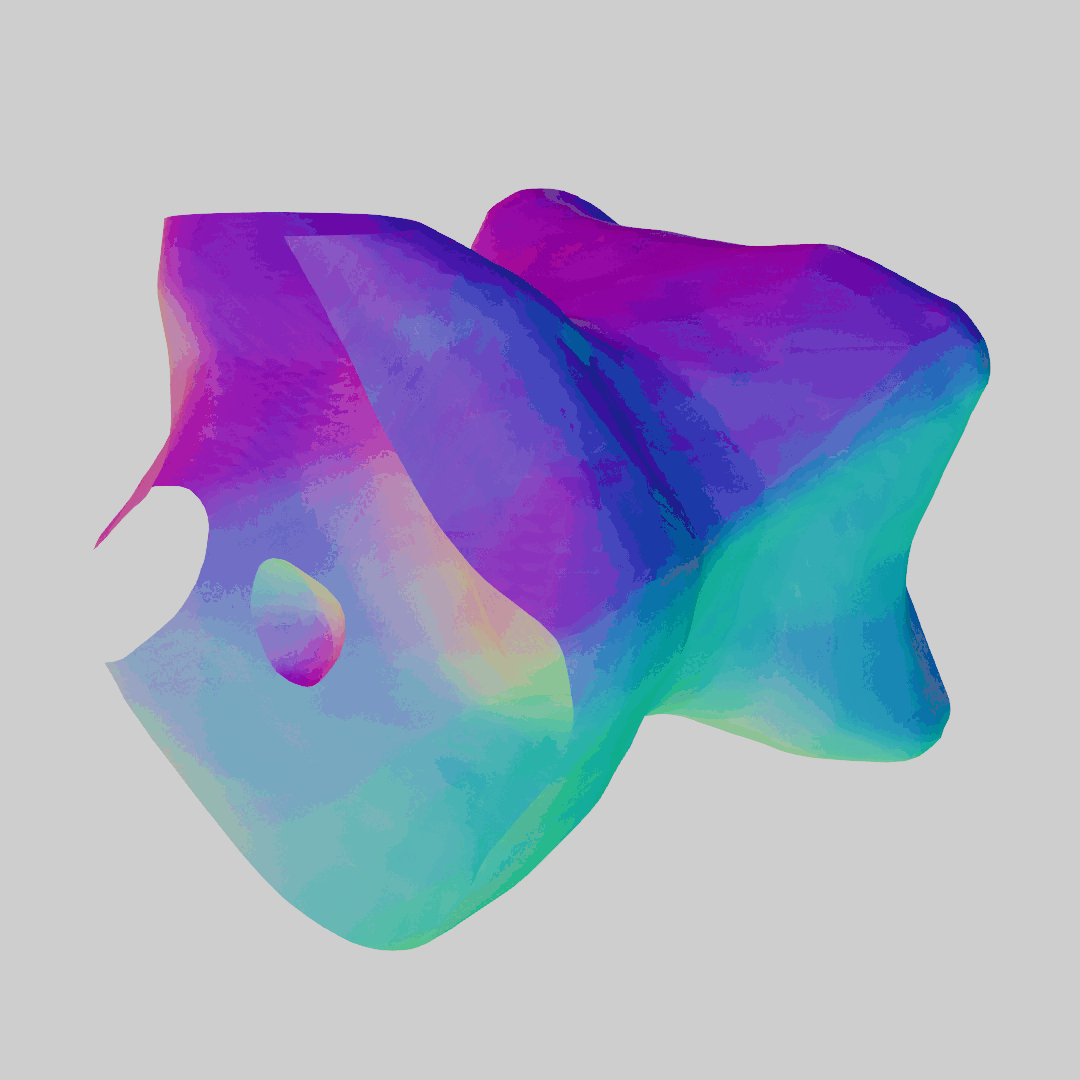Lerp from [1, 1] to [0, 0]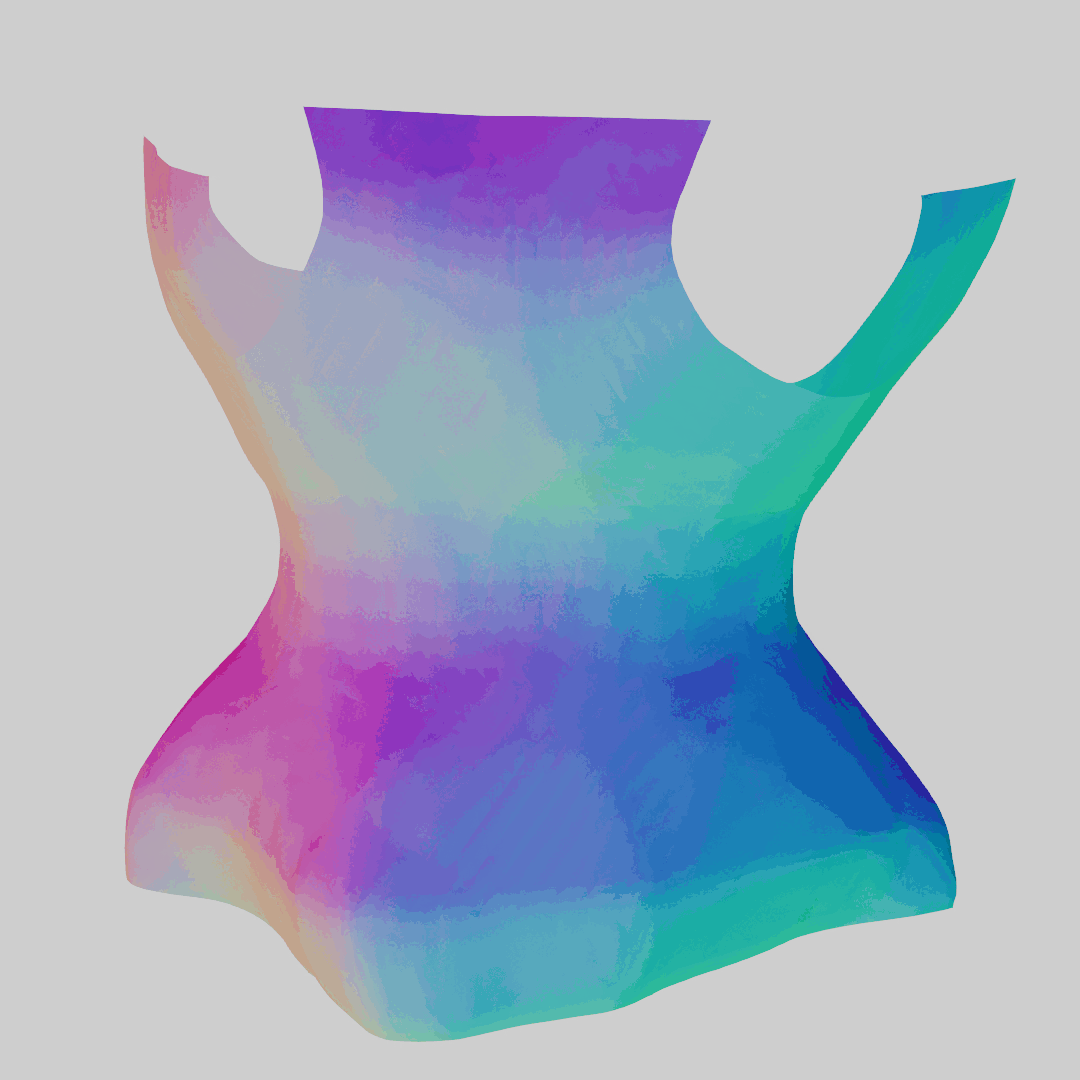Circular path

## Method 2: Classical Interpolation

In addition to a learning based approach, we also used classical interpolation techniques to interpolate between two meshes. Here, we start with the ground truth SDFs of two meshes. We sample these SDFs on a 64x64x64 voxel grid. The SDF values are then linearly interpolated between the two voxel grids. The voxel grids are converted into meshes via marching cubes. Below, we visualize some results. Notably, the learning based approach has fewer artifacts.